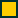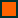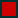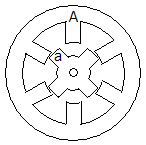Basic of SR MotorHow Torque is Produced in a Switched Reluctance Motor?

Positive torque in a SR motor is available at half the rotor pole pitch. When a rotor pole is unaligned with a stator pole, for example as shown in Figure 6a, energisation of Phase A enables the rotor to rotate until rotor pole 'a' aligns with stator pole 'A', Figure 6b. Positive torque is produced in this movement and the torque characteristic is similar to the characteristic as shown in Figure 5c.

Torque production in an SR motor can be explained based on energy conversion process. When one phase of the motor is energised, energy from the power supply is transferred to the phase winding. Part of the energy, which is also known as the co-energy, is used to produce mechanical movement. Torque produced in this movement is defined as the ratio of the change in co-energy to the change in rotor position. Co-energy at any rotor position is defined as the area under the flux linkage characteristic i.e. graph of flux linkage vs. current as shown in Figure 7. In mathematical expression, the torque production process can be summarised by the following equations:

Torque = DW'/Dq where W' is co-energy, q is rotor position

Co-energy = � y di where y is flux linkage, i is phase current

Figure 6a
Figure 6b
Fgure 7